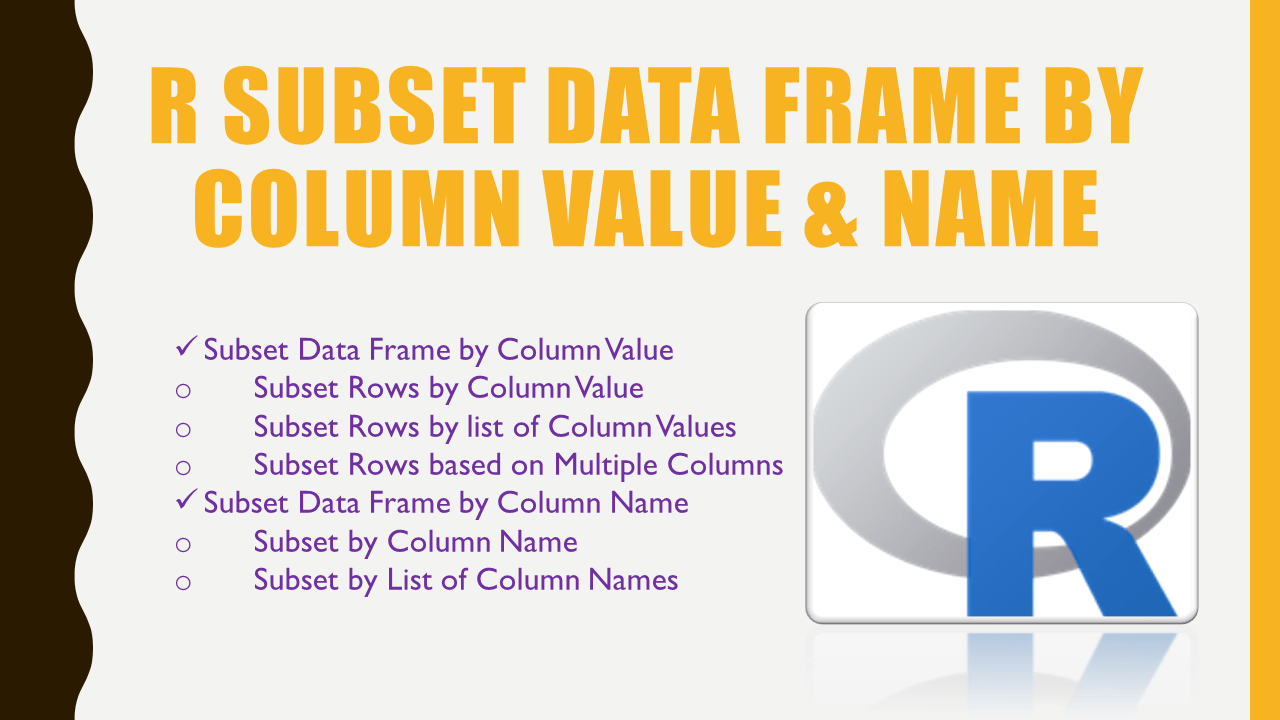# R Subset Data Frame by Column Value & Name

How to subset the data frame (DataFrame) by column value and name in R? By using R base df[] notation, or subset() you can easily subset the R Data Frame (data.frame) by column value or by column name.

## 1. Quick Examples of Subset DataFrame by Column Value & Name

Following are quick examples of how to subset the DataFrame to get the rows by column value and subset columns by column name in R.

``````
# Quick Examples

# Subset Rows by column value
df[df\$gender == 'M',]
subset(df, gender == 'M')

# Subset Rows by list of column Values
df[df\$state %in% c('CA','AZ','PH'),]
subset(df, state %in% c('CA','AZ','PH'))

# Subset Rows by Checking values on Multiple Columns
df[df\$gender == 'M' & df\$id > 15,]
subset(df, gender == 'M' & id >15)

# Subset DataFrame by column name id and name.
df[df\$gender == 'M', c('id','name')]
subset(df,gender == 'M',select = c('id','name'))
``````

Let’s create an R DataFrame, run these examples and explore the output. If you already have data in CSV you can easily import CSV file to R DataFrame. Also, refer to Import Excel File into R.

``````
# Create DataFrame
df <- data.frame(
id = c(10,11,12,13,14,15,16,17),
name = c('sai','ram','deepika','sahithi','kumar','scott','Don','Lin'),
gender = c('M','M','F','F','M','M','M','F'),
dob = as.Date(c('1990-10-02','1981-3-24','1987-6-14','1985-8-16',
'1995-03-02','1991-6-21','1986-3-24','1990-8-26')),
state = c('CA','NY',NA,NA,'DC','DW','AZ','PH'),
row.names=c('r1','r2','r3','r4','r5','r6','r7','r8')
)
df
``````

Yields below output.

``````
# Output
id    name gender        dob state
r1 10     sai      M 1990-10-02    CA
r2 11     ram      M 1981-03-24    NY
r3 12 deepika      F 1987-06-14  <NA>
r4 13 sahithi      F 1985-08-16  <NA>
r5 14   kumar      M 1995-03-02    DC
r6 15   scott      M 1991-06-21    DW
r7 16     Don      M 1986-03-24    AZ
r8 17     Lin      F 1990-08-26    PH
``````

## 2. Subset Data Frame by Column Value

### 2.1. Subset Rows by Column Value

Let’s use the R base square bracket notation `df[]` and subset() function to subset data frame rows based on a column value. The following example gets all rows where the column `gender` is equal to the value `'M'`. Note that the subset() takes the input data frame as first argument and the second should be condition you watned to apply.

``````
# Using df[]
df[df\$gender == 'M',]

# Using subset()
subset(df, gender == 'M')
``````

Yields below output.

``````
# Output
id  name gender        dob state
r1 16   Don      M 1986-03-24    AZ
r2 10   sai      M 1990-10-02    CA
r3 14 kumar      M 1995-03-02    DC
r4 15 scott      M 1991-06-21    DW
r5 11   ram      M 1981-03-24    NY
``````

### 2.2. Subset Rows by list of Column Values

By using the same options, you can also use an operator `%in%` to subset the DataFrame rows based on a list of values. The following example returns all rows where `state` values are present in vector values `c('CA','AZ','PH')`.

``````
# Using df[]
df[df\$state %in% c('CA','AZ','PH'),]

# Using subset()
subset(df, state %in% c('CA','AZ','PH'))
``````

Yields below output.

``````
# Output
id name gender        dob state
r1 16  Don      M 1986-03-24    AZ
r2 10  sai      M 1990-10-02    CA
r3 17  Lin      F 1990-08-26    PH
``````

### 2.3. Subset Rows based on Multiple Columns

If you wanted to check the conditions of multiple columns and subset the rows based on the result, use the below approach. In this example, I am using multiple conditions, each one with the separate column. This returns rows where `gender` is equal to M and `id` is greater than 15.

``````
# Using df[]
df[df\$gender == 'M' & df\$id > 15,]

# Using subset()
subset(df, gender == 'M' & id >15)
``````

Yields below output.

``````
# Output
id name gender        dob state
r1 16  Don      M 1986-03-24    AZ
``````

## 3. Subset Data Frame by Column Name

### 3.1 Subset by Column Name

Let’s use the same `df[]` notation and subset() function to subset the data frame by column name in R. To subset columns use `select` argument with values as column names to `subset()`.

``````
# Using df[]
df[df\$gender == 'M', 'id']

# Using subset()
subset(df,gender == 'M',select = 'id')
``````

### 3.2 Subset by List of Column Names

Similarly, let’s see how to subset the DataFrame by the list of column names in R. In order to have a list of column names, create a vector with the column names you are interested in using c() and assign this to the `select` argument. The following examples return the data frame with columns `id` and `name`.

``````
# Using df[]
df[df\$gender == 'M', c('id','name')]

# Using subset()
subset(df,gender == 'M',select = c('id','name'))
``````

Yields below output.

``````
# Output
id  name
r1 10   sai
r2 11   ram
r5 14 kumar
r6 15 scott
r7 16   Don
``````

## 4. Conclusion

In this article, you have learned how to subset data frame by column value and by column name in R. You can do this by using R base subset() or the square bracket notation df[].

## References

### Naveen (NNK)

Naveen (NNK) is a Data Engineer with 20+ years of experience in transforming data into actionable insights. Over the years, He has honed his expertise in designing, implementing, and maintaining data pipelines with frameworks like Apache Spark, PySpark, Pandas, R, Hive and Machine Learning. Naveen journey in the field of data engineering has been a continuous learning, innovation, and a strong commitment to data integrity. In this blog, he shares his experiences with the data as he come across. Follow Naveen @ @ LinkedIn